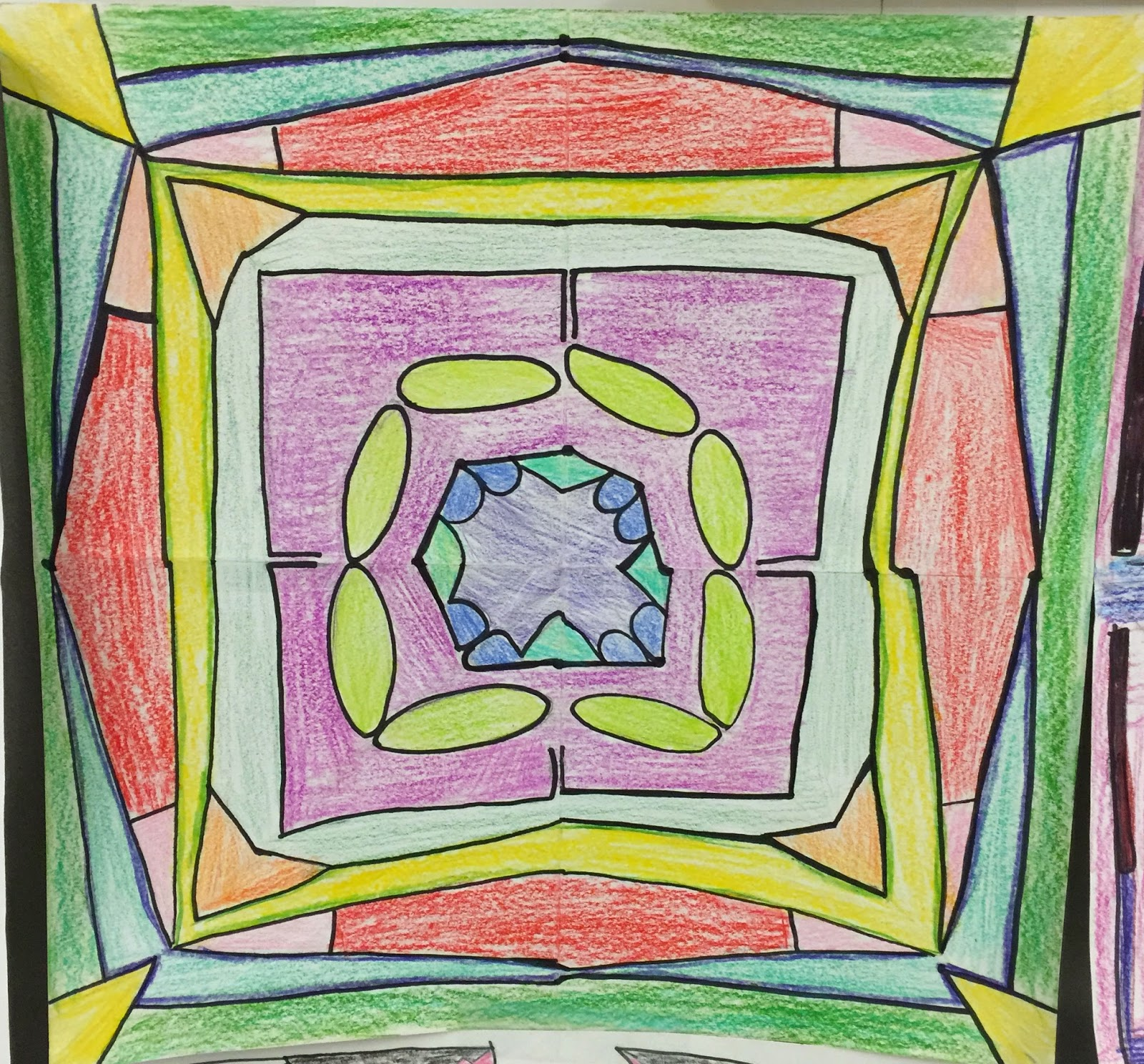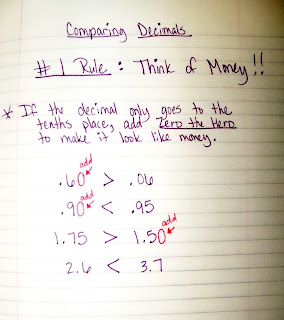Friday, March 3, 2017

Symmetrical NamesIf you look closely, you'll see Mrs.May on each sectionSarahTaylor is written on each sectionConnor
After our lesson on symmetry, we began making a symmetrical creation with our names. If you look closely, you'll see our names within the pictures. We folded a square sheet of paper into fourths, then folded that into a triangle. On each triangle, we wrote our names so they reflected the adjacent triangles. While they aren't perfect because they're drawn by hand, they turned out really cool! We had lots of discussions about the reflections as we went along...especially with the letter N. It's a confusing one! Some made their names go towards the middle, while others made theirs go towards the outside. Which ever direction they chose, they turned out great!

Tuesday, February 14, 2017

Comparing DecimalsProving Zero the Hero (in orange)
As we think about decimals, we really try to make them look like money. After all, the kids have been working with money since about 1st grade, and will continue to use it their whole lives! We began our lesson on comparing decimals by simply coloring pictures of different decimals. When we got to forty hundredths and four tenths, the kids noticed they both cover the same amount of space. After we wrote the decimals in fraction form, the kids could really see why they are equivalent - because 4/10 and 40/100 are equivalent fractions. This lead into our discussion of Zero the Hero! When our decimals only go the the tenths place, we can add him in the hundredths place and it doesn't change the value. He can help us see the decimal as money, and he will also help us as we begin adding and subtracting decimals next week!Adding Zero the Hero to make our decimal look like money

Friday, February 10, 2017

Decimal IntroductionClose-up of place value
As we introduced decimals, we talked about their relationship with fractions. Both are parts of a whole. We focused on our base 10 number system, and the pattern it follows. We always spend lots of time looking at picture examples, naming them in mathematical language, relating them to fractions, and labeling them. The biggest connection our kids make with decimals, is its relationship to money. We take lots of time to relate the tenths place to dimes, and the hundredths place to pennies.Decimal Anchor Chart

Friday, January 13, 2017

Complementary and Supplementary Angles

Today we looked at complementary and supplementary angles. Complementary angles are angles which add up to 90 degrees. Supplementary angles are angles which add up to 180 degrees. We can use what we know about these angles, as well as look at their adjacent angles, to determine missing angle measurements.

Wednesday, January 11, 2017

Types of Triangles

Today we learned about different types of triangles - right, acute, and obtuse. We discussed that all triangles have two acute angles. The third angle is what determines its type. If the third angle is also acute, it's an acute triangle. If the third angle is right, it's a right triangle. If the third angle is obtuse, it's an obtuse triangle.

Tuesday, January 10, 2017

Naming and Measuring Angles

We began the week naming angles. Most of the students were familiar with the types of angles, but we reviewed the important characteristics of straight angles, obtuse angles, acute angles, and right angles.

Next we worked on measuring angles. Measurement is still a very abstract idea for 4th graders, so we began by discussing measurement as a whole. What do we use different measurement tools to measure? I posed the question, "Would a ruler work when trying to measure angles?" In the beginning of our discussion, many kids believed it would. After trying it out, they came to the conclusion that we needed something that measures in degrees. They understood the unit wasn't correct, but weren't sure what degrees actually are, though we had discussed degrees when classifying angles as right, acute, obtuse, and straight.

We went back to everything we already know about angles. Going in a complete circle means we have gone 360 degrees, while only going halfway around means we have gone 180 degrees. We already know that a right angle is 90 degrees. We already know a straight angle is 180 degrees. We know an acute angle measures between 1 degree and 89 degrees. We know an obtuse angle measures between 91 and 179 degrees. Once we illustrated that, we decided we could half each 90 degree piece and figure out where 45 degrees and 135 degrees are located. Next we moved to

Monday, January 9, 2017

Lines and AnglesWe began lines and angles this week. We began with introductions of the different types of lines and angles, then moved to making them on dot paper. We moved on to looking at specific lines and angles within a large collection. This lead to the discussion of why it is important to have points on our lines - so we can name the ones we're specifically trying to reference.